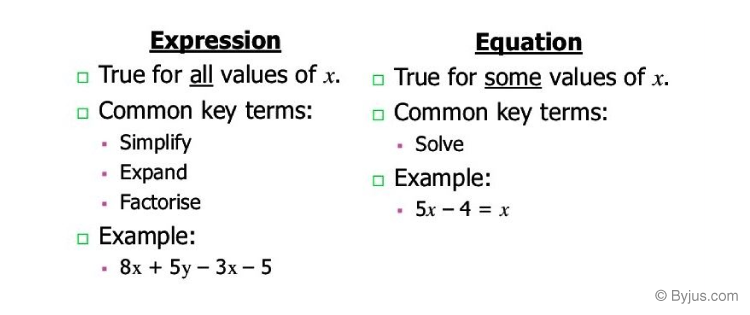# Algebra: Expressions And Equations

Mathematics is known as the “Queen of Science”. Algebra is a special branch of mathematics that deals with numbers, shapes and letters. We use concepts of mathematics everywhere, every day, almost in every situation. So far, we have used lots of numbers, lots of shapes and figures. Here, we are going to use some letters in math. Yes, it is algebra; let’s learn more about it.

Learn: Algebra## Expressions and Equations

Mathematics has been classified into many branches. Arithmetic is the branch that handles numbers and their operations. Arithmetic taught us addition, subtraction, multiplication and divisions of two or more numbers. Geometry is all about shapes and their construction using different tools like a compass, ruler and pencil. Algebra is another interesting branch where we express our daily life situations in numbers and letters (variables).

To understand algebra we need to know what an expression is and what an equation is.

## Expression Definition

An expression or algebraic expression is any mathematical statement which consists of numbers, variables and an arithmetic operation between them. For example, 4m + 5 is an expression where 4m and 5 are the terms and m is the variable of the given expression separated by the arithmetic sign +.

Variable is anything that varies; it doesn’t have a fixed value. Generally, expression variables are represented by alphabetical letters like a, b, c, m, n, p, x, y, z, and so on. We can form a variety of expressions using a combination of different variables and numbers.

Also, check: Expression Term Factor and Coefficient

### How to Simplify Algebraic Expressions?

The purpose of simplifying the algebraic expression is to find the simplified term of the given expression. To factorize or simplify the expression, we should first know how to combine the like terms, factor a number, order of operations. In combining like terms, the variables with the same degree are gathered together, and the constant terms are separated for the simplification process.

### Expression Examples

Few examples of expressions are as follows:

• x + 5y – 10
• 2x + 1
• x + y

## Equation Definition

The equation is an expression where two sides are connected through an equal sign (=). 2x + 1 = 9 is an equation, where 2x+1 is the left-hand side (LHS) and 9 is the expression’s right-hand side (RHS). The equal sign between LHS and RHS indicates that the value of LHS is equal to the RHS of the expression.

10x + 63 > 10, is not an equation. Here, the sign between LHS and RHS of the expression is not an equal sign. Hence, we can say every expression is not an equation. These kinds of expressions are called linear inequalities.

### Equation Examples

Few examples of equations are:

• 8m+5=10n
• a+4b = 12c+3

Note: An equation is interchangeable i.e. the equation remains the same even if LHS and RHS interchange each other.

### Difference between Algebraic Expression and Equation

 Expression Equation An expression is a number, a variable, or a combination of numbers and variables and operation symbols. An equation is made up of two expressions connected by an equal sign. Word example: The sum of 8 and 3 Word example: The sum of 8 and 3 is equal to 11. Expression: 8 + 3 Equation: 8 + 3 = 11 Expression with exponent: x2 – 4 Equation with exponent: x2 – 4 = 0

### How to Solve Algebraic Equations?

An algebraic equation contains two algebraic expressions separated by an equal sign (=) in between. The primary purpose of solving algebraic equations is to find the unknown variable in the given expression. While solving the equation, separate the variable terms on one side and constant terms on another side. The variable term can be isolated using the various arithmetic operations such as addition, subtraction, multiplication, division, and other operations like finding square roots, etc.

### Algebraic Expression and Equation Problem

Question: Find the value of x in the given equation: 4x + 10 = 30

Solution:

Given Equation: 4x + 10 = 30

Separate the variable and the constant term:

Keep the variable term on the left-hand side, and move the constant term on the right-hand side.

So, the given equation is written as:

4x = 30-10 [Performing subtraction operation]

4x = 20

x = 20/4 [Performing division operation]

x = 5.

Therefore, the value of x is 5.

Alternative Method:

Given equation is:

4x + 10 = 30

Subtracting 10 from both sides of the equation, we get;

4x + 10 – 10 = 30 – 10

4x = 20

Dividing both sides of equation by 4, we get;

4x/4 = 20/4

x = 5